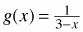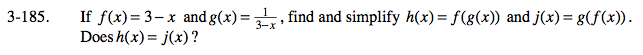### Home > CALC > Chapter 3 > Lesson 3.4.4 > Problem3-185

3-185.

If f(x) = 3 − x andfind and simplify h(x) = f(g(x)) and j(x) = g(f(x)). Does h(x) = j(x)? Homework Help ✎j(x) = ____________